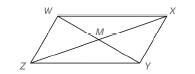Chapter 4.2, Problem 22EElementary Geometry For College St...

7th Edition
Alexander + 2 others
ISBN: 9781337614085

Solutions

Chapter
SectionElementary Geometry For College St...

7th Edition
Alexander + 2 others
ISBN: 9781337614085
Textbook Problem

In Exercise 19 to 24 , complete each proof. Given: ▱   W X Y Z with diagonals W Y ¯ and X Z ¯   ∆ W M X ≅ ∆ Y M Z Prove:To determine

To prove:

WMXYMZ

Explanation
 Given: ▱ WXYZ with diagonals WY¯ and XZ¯ ∆WMX≅∆YMZ Prove:

Still sussing out bartleby?

Check out a sample textbook solution.

See a sample solution

The Solution to Your Study Problems

Bartleby provides explanations to thousands of textbook problems written by our experts, many with advanced degrees!

Get Started

Evaluate 01(1x731x37)dx.

Calculus (MindTap Course List)

Expand each expression in Exercises 122. (2x3)(2x+3)

Finite Mathematics and Applied Calculus (MindTap Course List)

Find a formula for the inverse of the function. 24. y=x2x,x12

Single Variable Calculus: Early Transcendentals, Volume I

In problems 23-58, perform the indicated operations and simplify. 39.

Mathematical Applications for the Management, Life, and Social Sciences

8273

Applied Calculus for the Managerial, Life, and Social Sciences: A Brief Approach

Compute C7,7.

Understanding Basic Statistics

Which is the graph of ?

Study Guide for Stewart's Single Variable Calculus: Early Transcendentals, 8th